# Assignments

In the assignments below logarithmic equations must be solved. Note that a logarithm is defined for an argument greater than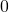. When the equation contains more than one logarithm all of these conditions have to be met (they define the so-called domain of the equation). When a solution is found it is valid if it lies in the domain.

1. Solve:Solution

2. Solvep: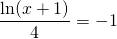Solution

3. Solve: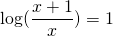Solution

4. Solve: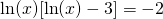Solution

5. Solve: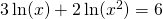Solution

6. Solve: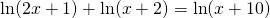Solution

7. Solve: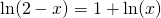Solution

8. Solve: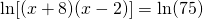Solution

9. Solve: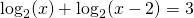Solution

10. Solve: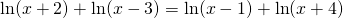Solution

0